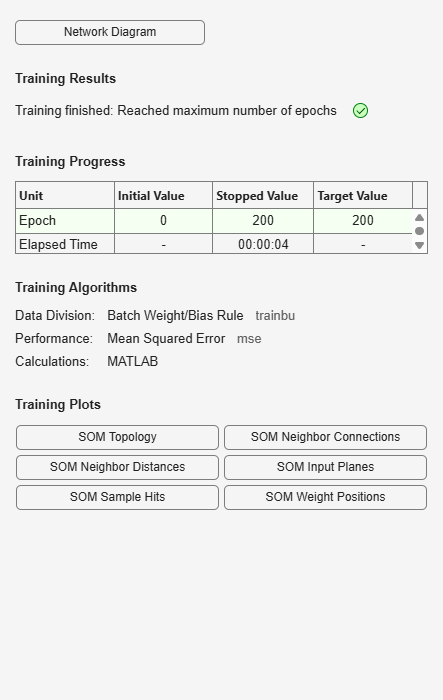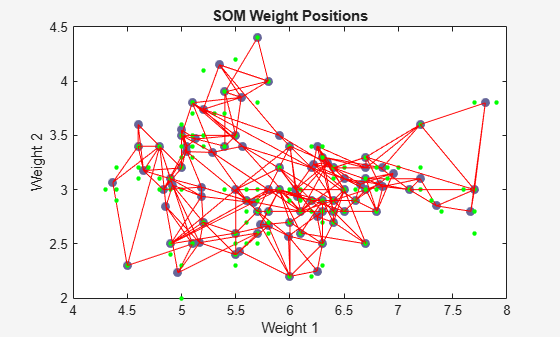# plotsompos

Plot self-organizing map weight positions

## Syntax

```plotsompos(net) plotsompos(net,inputs) ```

## Description

`plotsompos(net)` plots the input vectors as green dots and shows how the `SOM` classifies the input space by showing blue-gray dots for each neuron’s weight vector and connecting neighboring neurons with red lines.

`plotsompos(net,inputs)` plots the input data alongside the weights.

## Examples

### Plot SOM Weight Positions

```x = iris_dataset; net = selforgmap([10 10]); net = train(net,x);````plotsompos(net,x)`## Version History

Introduced in R2008a